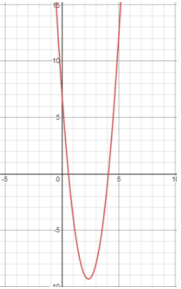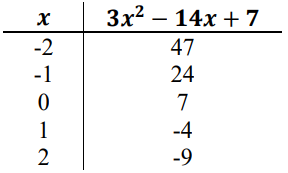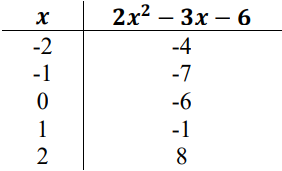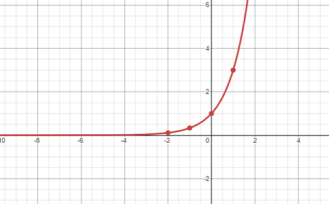# MA.912.F.3.6Export Print
Determine whether an inverse function exists by analyzing tables, graphs and equations.
General Information
Subject Area: Mathematics (B.E.S.T.)
Strand: Functions
Status: State Board Approved

## Benchmark Instructional Guide

### Terms from the K-12 Glossary

• Composition of functions
• Inverse of a function

### Vertical Alignment

Previous Benchmarks

Next Benchmarks

### Purpose and Instructional Strategies

In Algebra I, students analyzed linear, quadratic, exponential and absolute value functions. In Mathematics for College Algebra, extend their knowledge of analyzing functions to determine whether an inverse function exists by analyzing tables, graphs, and equations (MTR.2.1)
• Students will need to use what they have learned about key features and characteristics and patterns that are evident with each function type to determine whether the functions they see represented in graphs, tables, and/or equations are functions that are invertible (MTR.5.1)
• When dealing with a graph, students can use the horizontal line test to determine if an inverse to the function exists. The horizontal line test allows a student to check if a function is one-to-one, meaning there is only one $x$-value for each $y$-value. In order to use the horizontal line test, students can draw a horizontal line and check to see if it intersects the graph of the function in all places at exactly one point. If it does, then the given function should have an inverse that is also a function.
• Instruction includes discussing what adjustments could be made to the function to make it where an inverse to the function will exist (MTR.5.1)
• Instruction includes using compositions of functions to determine whether two functions are inverses of each other (MTR.2.1).

### Common Misconceptions or Errors

• Students may confuse the horizontal line test (used to determine if the function has an inverse) with the vertical line test which determines if the graph represents a function.
• Students may not understand how to transpose the input and output values in a table to determine the inverse of a function.
• Students may not understand that it is possible for a function to have an inverse that may not be a function.

• Create a function that has an inverse. Explain using a table, graph, and equation how you know that an inverse exists.

• Create a function for which an inverse does not exist. Explain what about that function makes it not invertible. Explain what adjustments could be made to the function to make it invertible.

• Determine whether an inverse function exists for the function given below in the graphical and numerical representations. Explain what features you found in the graph as well as the table that helped you determine whether the function had an inverse or not.### Instructional Items

Instructional Item 1
• Determine whether an inverse to the following function represented in the table exists. Explain.Instructional Item 2
• Determine whether an inverse to the following function represented by the following graph exists. Explain.Instructional Item 3
• Determine whether an inverse to the following function represented with the equation exists. Explain.
$f$($x$) = ln ($x$)

*The strategies, tasks and items included in the B1G-M are examples and should not be considered comprehensive.

## Related Courses

This benchmark is part of these courses.
1200330: Algebra 2 (Specifically in versions: 2014 - 2015, 2015 - 2022, 2022 and beyond (current))
1200340: Algebra 2 Honors (Specifically in versions: 2014 - 2015, 2015 - 2022, 2022 and beyond (current))
1200400: Foundational Skills in Mathematics 9-12 (Specifically in versions: 2014 - 2015, 2015 - 2022, 2022 and beyond (current))
7912095: Access Algebra 2 (Specifically in versions: 2016 - 2018, 2018 - 2019, 2019 - 2022, 2022 and beyond (current))
1200710: Mathematics for College Algebra (Specifically in versions: 2022 and beyond (current))

## Related Access Points

Alternate version of this benchmark for students with significant cognitive disabilities.
MA.912.F.3.AP.6: Determine whether an inverse function exists by analyzing graphs and equations.

## Related Resources

Vetted resources educators can use to teach the concepts and skills in this benchmark.

## Student Resources

Vetted resources students can use to learn the concepts and skills in this benchmark.

## Parent Resources

Vetted resources caregivers can use to help students learn the concepts and skills in this benchmark.